## Load Graph

Table Time Chart Windows Overview

Menu Bar Window

The load graph is a table time chart that shows load on each resource. This graph shows how much load is put on each resource in graphical and numerical format.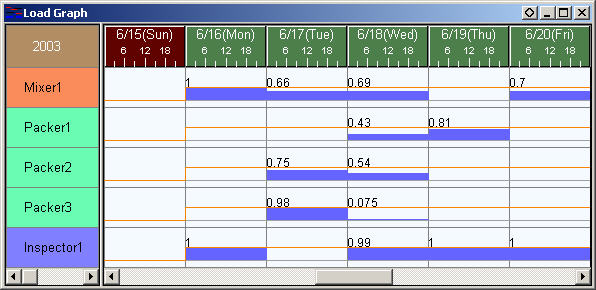Chart content

There are two types of graphs for the load graph, the "Load ratio graph" and "Load amount graph". The load ratio graph is displayed by default but you can also display the load amount graph by changing the setting of the property "Resource row content" in the Chart content tab of the display settings to "Load amount graph".

The load ratio graph shows the average percentage by which the resource quantity specified in the calendar table is used, while the load amount graph shows the average resource quantity that is used.
On the other hand, the Load graph represents the average resource quantity that is used.

The specific calculations are as follows.
A value in each cell of the load ratio graph is [resource qty time actually assigned to the resource as a use instruction] / [resource qty time given in the calendar settings] of the corresponding period. A value in each cell of the load amount graph is [resource qty time actually assigned to the resource as a use instruction] of the corresponding period.

The "resource qty time" here means the total resource quantity per second in the summary period divided by the summary period (in seconds), i.e. the resource quantity used per unit time.

Note that, in versions earlier than Ver. 15.2, this is set in the “Number Format” property for both load ratio graphs and load amount graphs, but when you select the "Load amount graph" for the property "Resource row content", we recommend changing the property "Number format" in the Load graph tab from
Format(ME.ASGLoadValue_Value,'0%')
to
Format(ME.ASGLoadValue_Value,'0.00')
so as not to show the "%" sign.
In Ver. 15.2 and later, specify the display text in the "Load Factor Graph Character Expression" and "Load Amount Graph Character Expression" properties, respectively.

Start time of summary period (start day of week, start day of month) ( Ver.13 restricted features. )

When tabulating on a weekly basis, the start day of the week can be set in the "Start day of week” property. By default, month is set, and weekly summary is done with Monday as the start day of the week.
Normally, this setting is done in accordance with the setting of the “Start day of week” property on the time axis of the time chart.

When tabulating on a monthly basis, the start day of the month can be set in the "Start day of month” property. By default, the 1st is set, and monthly summary is done for the 1-month period starting from the 1st of the month. If the 20th is set, monthly summary is done from the 20th of the month until the 19th of the next month.
Normally, this setting is done in accordance with the setting of the “Start day of month” property on the time axis of the time chart.

Graph coloring

The default settings for coloring of the load graph are to show loads of 100% in blue, and loads exceeding 100% in red. (Prior to Ver.6.0.2 the load value for changing these colors was 200%)The colors for these displays can be changed by setting the 'Display color expression' of the chart properties (feature added from Ver.6.0.2).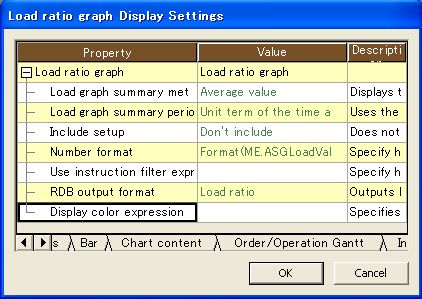The following expression found in the standard registered expressions for this property:

If(ME.Value<0.8,RGB(0,255,0),If(ME.Value<=1.0,RGB(255,255,0),RGB(255,0,0)))

can be set to obtain a display as in the image below, where bars are green for load less than 80%, yellow for load between 80% and 100%, and red for any higher load.
Since this is an expression, it allows for a wide variety of possibilities for different color display schemes.

Regardless of the display color expression, when the load exceeds 200% a red bar will be shown above the resource row.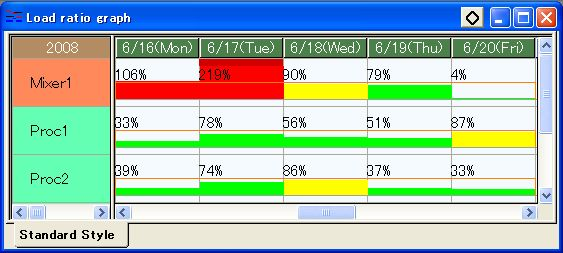Use instruction filtering

The 'Use instruction filter expression' property in the load graph display settings can be used to display the portion of load caused by certain use instructions in a different color.

* The ability to display a subset of use instructions in a separate color was added from Ver.6.1.2. In previous versions, this filter expression would simply not display use instructions that did not meet the filter criteria.

The upper part of the image below is using the filter expression

ME.Work.Type=='P'&&ME.Order.Type!='E'

which filters to only production tasks (this can be found in the standard registered expressions).
The production task portion of the bar is shown in yellow, and load from any other task type is shown in blue.
The lower part of the image is not using any use instruction filter expression. The whole bar is displayed in the standard blue color.

The values for the whole load and the filtered portion of the load can also be obtained and displayed. In the images below, the expression

Format(ME.UnfilteredValue,'Overall = 0%')+'\n '+Format(ME.Value,'Filtered = 0%')

is set as the bar text display expression (this is also a standard registered expression).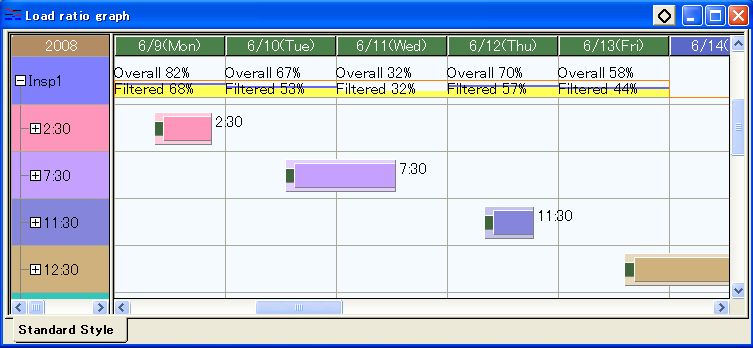The color used to display these filtered parts of the bar can be changed by customizing display colors. The default color is yellow.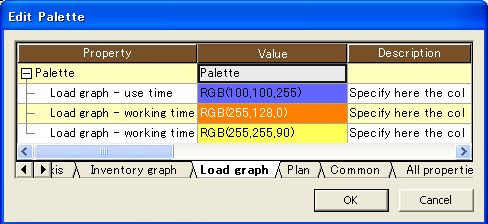### Status bar text

The status bar for the load graph shows the resource, time, resource quantity of the filtered tasks, and the total resource quantity for the cell which the mouse is over.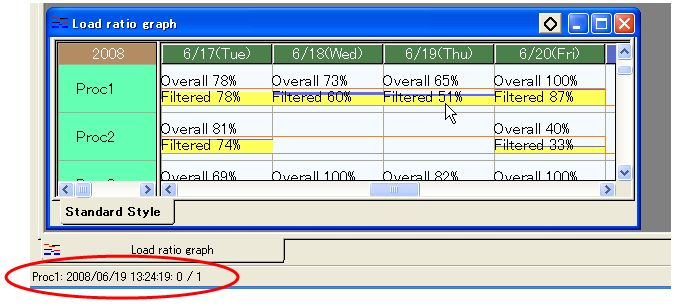### Group display

For rows which are actually resource groups, the load shown is the total load of all resources in the group.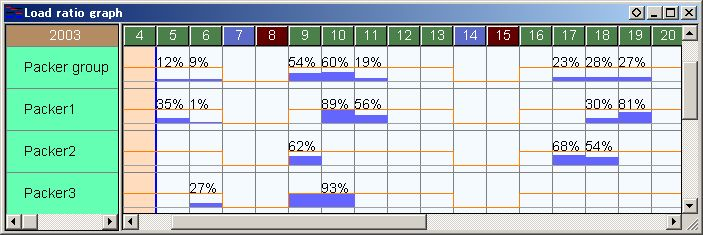### Left part text expressions

Resources are shown on the left side of load graph (vertical axis).
With resource left part text expression property found from resource Gantt chart display settings (table tab), the contents of the information shown on left can be changed.

The above mentioned property setting is reflected to other charts such as operation chart and pegged order chart as well.<Example>Code + name
Me+"\n "+ Me.Name

### Background color expression on the left part (Ver.8 restricted feature)

There is a tab to set the left part of the Load Graph in the tab named Chart display settings.
By Resource left part color expression
it can be done.
Versions before 7.3.1, the color can be changed.

<Example>Color of resource group
Res_Group.Res_Color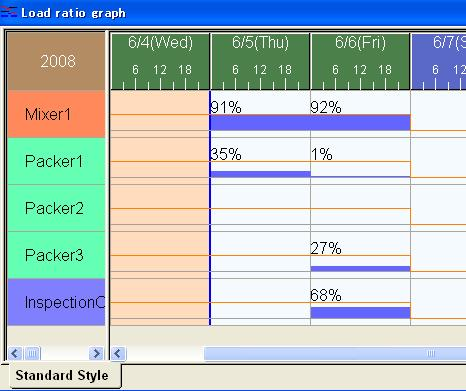### Menu

In a load graph, you can perform various operations using the [Chart] menu available in the Menu.

### Popup menu

Right-clicking the mouse on each area of a load graph invokes a corresponding popup menu.